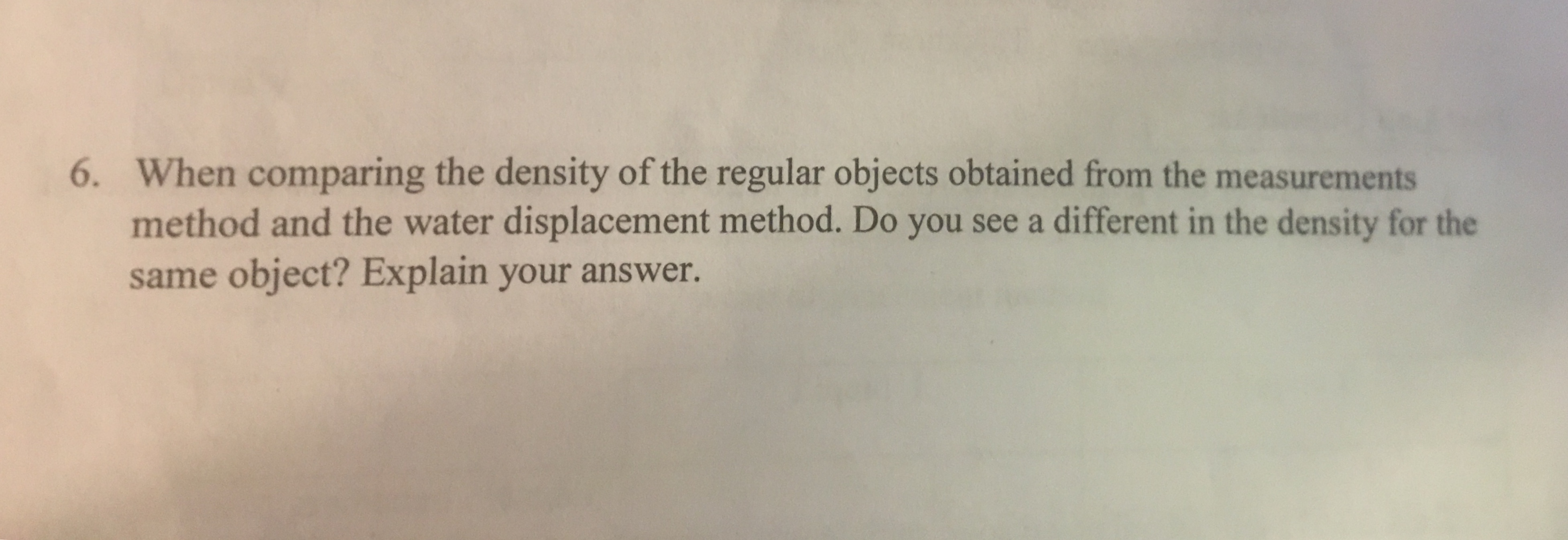# 6. When comparing the density of the regular objects obtained from the measurementsmethod and the water displacement method. Do you see a different in the density for thesame object? Explain your answer.

Question
3 viewshelp_outlineImage Transcriptionclose6. When comparing the density of the regular objects obtained from the measurements method and the water displacement method. Do you see a different in the density for the same object? Explain your answer. fullscreen
check_circle

Step 1

Density:

Density is the measure of how much matter occupies a given amount of space. It is quantified with the ratio of mass (m) per unit volume (V).

Step 2

Determining of volume by measurement methods:

First, we have to measure all the dimensions of an object using any measuring device like Vernier caliper etc. Then we have to calculate the volume of object depending on our measurements.

In this method of measurement, density calculated will depend on accuracy of our measurements. if measurements are accurate then we will get accurate value of density.

Step 3

Determining Volume with Water Displacement:

Volume is a measure of the amount of space an object takes up.  When an object is submerged in water, it pushes water out of the way.  If we measure the amount the water level increases...

### Want to see the full answer?

See Solution

#### Want to see this answer and more?

Solutions are written by subject experts who are available 24/7. Questions are typically answered within 1 hour.*

See Solution
*Response times may vary by subject and question.
Tagged in

### General Chemistry